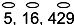#### You may also like### Tweedle Dum and Tweedle Dee

Two brothers were left some money, amounting to an exact number of pounds, to divide between them. DEE undertook the division. "But your heap is larger than mine!" cried DUM...### Sum Equals Product

The sum of the numbers 4 and 1 [1/3] is the same as the product of 4 and 1 [1/3]; that is to say 4 + 1 [1/3] = 4 ï¿½ 1 [1/3]. What other numbers have the sum equal to the product and can this be so for any whole numbers?### Special Sums and Products

Find some examples of pairs of numbers such that their sum is a factor of their product. eg. 4 + 12 = 16 and 4 × 12 = 48 and 16 is a factor of 48.

# Egyptian Fractions

##### Age 11 to 14Challenge Level

Egyptian Fractions printable sheet

The ancient Egyptians didn't write fractions with a numerator greater than 1 - they wouldn't, for example, write $\frac{2}{7}$, $\frac{5}{9}$, $\frac{123}{467}$.....

Instead they wrote fractions like these as a sum of different unit fractions.

There are several NRICH problems based on Egyptian fractions. You can start by exploring unit fractions at Keep it Simple

In this problem we are going to start by considering how the Egyptians might have written fractions with a numerator of 2 (i.e. of the form $\frac{2}{n}$).
For example

$\frac{2}{3} = \frac{1}{3} + \frac{1}{3}$ (but since these are the same, this wasn't allowed.)
or
$\frac{2}{3} = \frac{1}{3} + \frac{1}{4} + \frac{1}{12}$
or
$\frac{2}{3} = \frac{1}{3} + \frac{1}{5} + \frac{1}{20} + \frac{1}{12}$
or
$\frac{2}{3} = \frac{1}{3} + \frac{1}{6} + \frac{1}{30} + \frac{1}{20} + \frac{1}{12}$
or
$\frac{2}{3} = \frac{1}{4} + \frac{1}{12} + \frac{1}{7} + \frac{1}{42} + \frac{1}{31} + \frac{1}{930} + \frac{1}{21} + \frac{1}{420} + \frac{1}{13} + \frac{1}{156}$
and so on, and so on!!

You might want to check that these are correct.
(If you can't see how these have been generated, take a look at Keep it Simple )

#### BUT wouldn't it be simpler to write it as the sum of just two different unit fractions?

For $\frac{2}{3}$ that's quite easy.........$\frac{2}{3} = \frac{1}{2} + \frac{1}{6}$

But is it always so easy?
Try some other fractions with a numerator of 2.
Can they also be written as the sum of just two different unit fractions?

#### Can all fractions with a numerator of 2 (i.e. of the form $\frac{2}{n}$) be written as the sum of just two different unit fractions?

Can you find an efficient method for doing this?

You might want to explore fractions of the form $\frac{3}{n}$, $\frac{4}{n}$, $\frac{5}{n}$...... and think about how the Egyptians would have represented these, using sums with the least number of unit fractions.

You might like to take a look at a follow up problem, The Greedy Algorithm

NOTES AND BACKGROUND

The ancient Egyptians lived thousands of years ago, how do we know what they thought about numbers? A little research on this topic will show that famous mathematicians have asked and answered questions about the Egyptian fraction system for hundreds of years. You can find references to results in this field that were proved in the 1200s and in the 2000s, and you can also find some open questions - things mathematicians think are true, but have not been proved yet.

Throughout history, different civilisations have had different ways of representing numbers. Some of these systems seem strange or complicated from our perspective. The ancient Egyptian ideas about fractions are quite surprising.

For example, they wrote $\frac{1}{5}$, $\frac{1}{16}$ and $\frac{1}{429}$ as(but using their numerals)

They didn't write fractions with a numerator greater than 1 - they wouldn't, for example,write $\frac{2}{7}$, $\frac{5}{9}$, $\frac{123}{167}$.... although there is evidence that the specific fraction $\frac{2}{3}$ was used by the Egyptians, and $\frac{3}{4}$ sometimes as well. They had special symbols for these two fractions.

The Rhind Mathematical Papyrus is an important historical source for studying Egyptian fractions - it was probably a reference sheet, or a lesson sheet and contains Egyptian fraction sums for all the fractions $\frac{2}{3}$, $\frac{2}{5}$, $\frac{2}{7}... \frac{2}{101}$.
Why did they only include the odd ones?

#### $\frac{4}{n}$ and $\frac{3}{n}$

In the 1940s, the mathematicians Paul Erdos and Ernst G. Straus conjectured that every fraction with numerator = 4 can be written as an Egyptian fraction sum with three terms. If you have found an example that appears to need more than three, can you find an alternative sum? Can you find a reason why it must work, or a counter-example - the conjecture isn't yet proved. It is proved for $\frac{3}{n}$.